Next: The Local Group and Up: Formation of Large-scale Structures Previous: Generation of Perturbations

# Peculiar Velocity Fields

In a perfectly homogeneous Friedmann-universe, comoving particles will have velocities solely determined by the Hubble law. By introducing inhomogeneities, as in the standard model, the Hubble flow will be perturbed, resulting in peculiar velocities of the particles. These velocities can give us valuable information about the underlying mass distribution, and vice versa.

A discussion of the velocity fields can be found in textbooks like P-93 and Pad.

The peculiar velocities of galaxies emerge from the linear theory discussed in Subsection (2.2.2). The linearized mass conservation equation in comoving coordinates is given by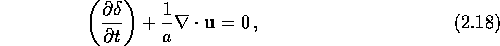where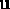is the peculiar velocity and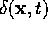is the density contrast (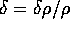). The calculations are greatly simplified by transferring this equation into Fourier space, where one can treat each wavelength separately. The Fourier transform of the density contrast and the peculiar velocity are, respectively: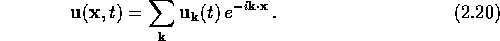By inserting these two equations into equation (2.18), and rearranging somewhat, the resulting peculiar velocities are(A term has been ignored since it decreases with time.)can be rewritten as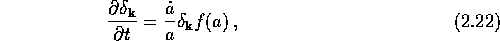where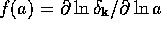. By combining equation (2.22) and (2.21), one have an expression for the relation between velocity and density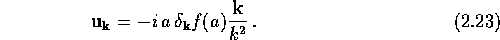The value of f(a) at the current epoch has been shown to be very well approximated by the power law[for further details, see][page 163]Pad. Using this value for f, one sees that the density parameter can be estimated if the velocity field and the density field were observationally determined. The problem is to get an accurate enough determination of the velocity field, which involve distance determinations which are not based on redshift.

In the gravitational instability picture one assumes that it is gravity that makes the perturbations grow. It is therefore natural to introduce the gravitational potential instead of the density contrast. The linearized Poisson equation in comoving coordinates is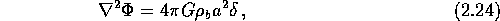where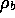is the background density. Solving for the Fourier transform density contrast gives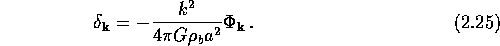Inserting this into equation (2.23) results in an expression for the connection between the velocity field and the gravitational potentialEquation (2.26) does not do us much good in the Fourier space--let us transfer it into real space:For a particle system, with masses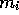, this equation can be rewritten asThis equation show us that the velocity field is parallel and proportional to the peculiar acceleration, and then also to the gravitational force.Next: The Local Group and Up: Formation of Large-scale Structures Previous: Generation of Perturbations

Trond Hjorteland
Mon Jul 5 02:59:28 MET DST 1999